Fibre space

(diff) ← Older revision | Latest revision (diff) | Newer revision → (diff)

An object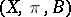, whereis a continuous surjective mapping of a spaceonto a space(a fibration).,andare also called the total space, the base and the projection of the fibre space, respectively, and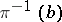is called the fibre above. A fibre space can be regarded as the union of the fibres, parametrized by the baseand glued by the topology of. For example, there is the product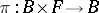, whereis the projection onto the first factor; the fibration-base, where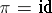andis identified with; and the fibre space over a point, whereis identified with a (unique) space.

A section of a fibration (fibre space) is a continuous mappingsuch that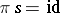.

The restriction of a fibration (fibre space)to a subset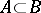is the fibration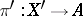, whereand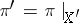. A generalization of the operation of restriction is the construction of an induced fibre bundle.

A mapping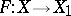is called a morphism of a fibre space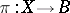into a fibre space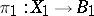if it maps fibres into fibres, that is, if for each point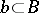there exists a pointsuch that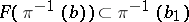. Such andetermines a mapping, given by.is a covering of, and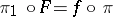; the restrictionsare mappings of fibres. If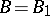and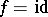, thenis called a-morphism. Fibre spaces and their morphisms form a category containing the fibre spaces overand their-morphisms as a subcategory.

Any section of a fibrationis a fibre space-morphism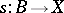from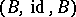into. If, then the canonical imbeddingis a fibre space morphism from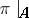to.

Whenis a homeomorphism, it is called a fibre space isomorphism, a fibre space isomorphic to a product is called a trivial fibre space, and an isomorphism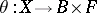is called a trivialization of.

If each fibreis homeomorphic to a space, thenis called a fibration with fibre. For example, in any locally trivial fibre space over a connected base, all the fibresare homeomorphic, and one can taketo be any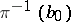; this determines homeomorphisms.

Both the notationsandare used to denote a fibration, a fibre space or a fibre bundle.
In the West a mappingwould only be called a fibration if it satisfied some suitable condition, for example, the homotopy lifting property for cubes ( "Serre fibration" ; see Covering homotopy for the homotopy lifting property, [a3]). A mappingwould be called a morphism (respectively, an isomorphism) only if the induced functionwere continuous (respectively, a homeomorphism).